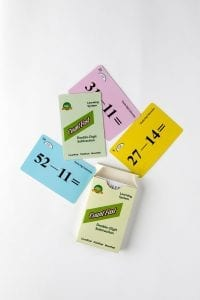# Double-Digit Subtraction Home Activities

####OVERVIEW & PURPOSE

Week five of the 2nd Grade CountFast program focuses the general strategies for subtracting double-digit numbers with quick mental calculation.  (Subtraction with ‘borrowing’ or ‘trading in’, and subtraction with no borrowing.)  Card decks should go home with students each day for additional practice with a parent at home. Each week, a new deck is introduced and the previous deck is for the student to keep at home for continued practice.

#### EDUCATION STANDARDS

1. NCTM Standard: develop fluency with basic number combinations for addition and subtraction
2. NCTM Standard: understand the effects of adding and subtracting whole numbers
3. CCSS.MATH.CONTENT.2.NBT.B.5
Fluently add and subtract within 100 using s
1. Develop strategies for fluency in solving double-digit subtraction equations by understanding the process of ‘borrowing’ or ‘trading in’ when needed.
DAY 1:Have your child use the YELLOW cards in the deck to explain how to quickly subtract double-digit numbers when there is no need to ‘borrow’ or ‘trade in’.  Students turn over one card at a time and solve the problem as fast as they can, being sure to explain to you how he/she arrived at the answer.  For example, 25 – 13 can be explained in this way: “Twenty-five take away 13 is 12.  I have enough ones and tens in the original number, so I did not need to borrow or trade.”  Of course, there are other methods students can use for quick mental calculation.  Some students may find it faster to decompose the numbers and subtract those smaller parts. (25 -13 can be thought of as 25 – 10 – 3).  Encourage your child to use the fastest mental calculation method for his/her way of thinking.DAY 2:

Have your child use the BLUE cards in the deck to explain how to quickly subtract double-digit numbers in which the answer will end in a zero. Guide them to notice that on each blue card, the answer when subtracting the digits in the one’s place equals zero.  This makes it quick and easy to subtract the numbers in the ten’s place and add a zero on the end to get the final difference.  For example, 31 – 11 can be solved quickly by noticing that the one’s digits (1 – 1) will equal 0.  This just leaves subtraction of the ten’s digits (3 – 1 which equals 2) and then add a 0 after that answer (2) to get 20 as a final difference.

DAYS 3 through 5:

Have your child use the GREEN/GOLD cards in the deck to explain how to quickly calculate double-digit subtraction problems that require ‘borrowing’ or ‘trading in’ from the ten’s place. Review the subtraction concept of the top (or first) number being how much we HAVE to start with.  The bottom (or second) number is what we are taking AWAY.  Sometimes there are not enough ones in the top (or first) number to subtract the bottom (or second) number.  AND – we know that Commutative Property does not work for subtraction, so we cannot just transpose the numbers to make it ‘easier’.  Review borrowing or trading in from the tens group to look at the original number differently without changing its value. (42 – 29 requires a trade-in because we cannot take 9 ones away from 2 ones.  We must rewrite 42 as 3 tens and 12 ones in order to subtract.)

Some children will look at the problems differently (such as 42 – 29 can be solved by subtracting 20 from 42, then subtracting 9 more; OR subtracting 30 from 42, then adding one back on).  Encourage your child to use a method that is FASTEST for the way he/she thinks.

**For additional fun, family members can ‘race’ each other to see who can mentally calculate the answers to a particular color set of cards (or ALL of the cards) the fastest!  Who will win??  What strategies will you use?

Stay SharpFree flash card decks to keep kids entertained and sharp!

To help you keep your kids math sharp during this crisis, we're giving you 3 free CountFast flash card decks for just \$6 in shipping.  Just have them take the quick quiz for their grade at the link below and you'll get a discount code to use on any three decks.